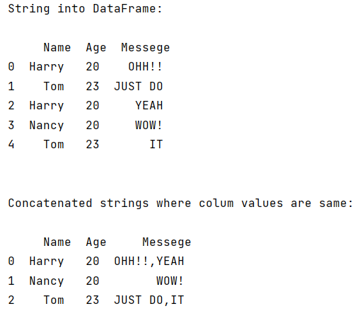# Concatenate strings from several rows using pandas groupby

Given a Pandas DataFrame, we have to concatenate strings from several rows using pandas groupby. By Pranit Sharma Last updated : September 17, 2023

Pandas is a special tool which allows us to perform complex manipulations of data effectively and efficiently. Inside pandas, we mostly deal with a dataset in the form of DataFrame. DataFrames are 2-dimensional data structure in pandas. DataFrames consists of rows, columns and the data.

A string in pandas can also be converted into pandas DataFrame with the help StringIO method.

## Problem statement

Given a Pandas DataFrame, we have to concatenate strings from several rows using pandas groupby. Suppose we have a product name ‘Frooti’ in our DataFrame and its sales in number. We may have this product multiple times in the DataFrame.

## Concatenating strings from several rows using pandas groupby

For this purpose, we will use the df.groupby() method by passing the set of the columns whose value we have to concatenate and a new column name. Also, we will apply the lambda expression with the .join() method. Consider the following code statement to achieve this task:

```df.groupby(['Name','Age'])['Messege'].apply(lambda x: ','.join(x)).reset_index()
```
Note

To work with pandas, we need to import pandas package first, below is the syntax:

```import pandas as pd
```

To work with StringIO, we need to import io package from StringIO first, below is the syntax:

```from io import StringIO
```

Let us understand with the help of an example,

## Python program to concatenate strings from several rows using pandas groupby

```# Importing pandas package
import pandas as pd

# Importing StringIO module from io module
from io import StringIO

# Creating a string
string= StringIO("""
Name;Age;Messege
Harry;20;OHH!!
Tom;23;JUST DO
Harry;20;YEAH
Nancy;20;WOW!
Tom;23;IT
""")

# Reading String in form of csv file

# Printing the DataFrame
print("String into DataFrame:\n\n",df,"\n\n")

# Applying function to concatenate strings
result = df.groupby(['Name','Age'])['Messege'].apply(lambda x: ','.join(x)).reset_index()

# Display result
print("Concatenated strings where colum values are same:\n\n",result)
```

### Output

The output of the above program is: